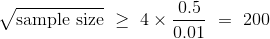# 十二、为什么均值重要

• 均值正好测量了什么？
• 大部分数据与平均值有多接近？
• 样本量如何与样本的均值相关？
• 为什么随机样本的经验分布出现钟形？
• 我们如何有效地使用抽样方法进行推理？

## 均值的性质

`np.average``np.mean`方法返回数组的均值。

``````not_symmetric = make_array(2, 3, 3, 9)
np.average(not_symmetric)
4.25
np.mean(not_symmetric)
4.25
``````

### 基本性质

• 它不一定是集合中的一个元素。
• 即使集合的所有元素都是整数，也不一定是整数。
• 它在集合的最小值和最大值之间。
• 它不一定在两个极值的正中间；集合中一半的元素并不总是大于均值。
• 如果集合含有一个变量的值，以指定单位测量，则均值也具有相同的单位。

### 均值的性质

``````zero_one = make_array(1, 1, 1, 0)
sum(zero_one)
3
np.mean(zero_one)
0.75
``````

``````np.mean(make_array(True, True, True, False))
0.75
``````

### 均值和直方图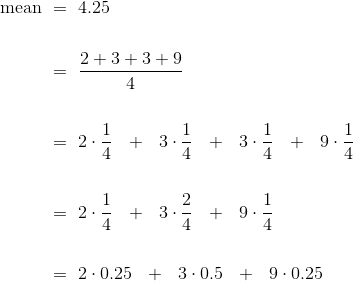``````not_symmetric
array([2, 3, 3, 9])
same_distribution = make_array(2, 2, 3, 3, 3, 3, 9, 9)
np.mean(same_distribution)
4.25
``````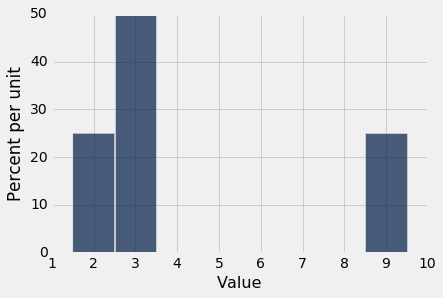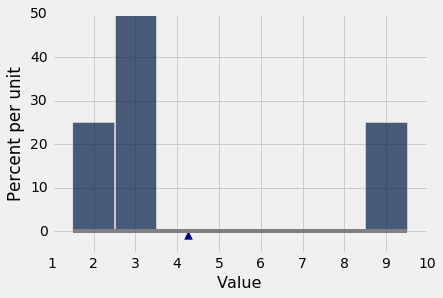### 均值和中位数

``````symmetric = make_array(2, 3, 3, 4)
``````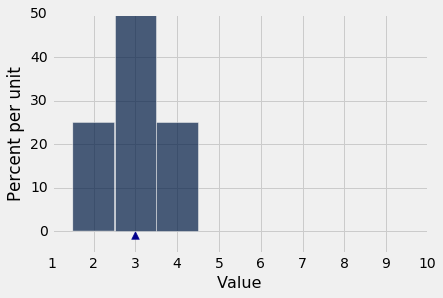``````np.mean(symmetric)
3.0
percentile(50, symmetric)
3
``````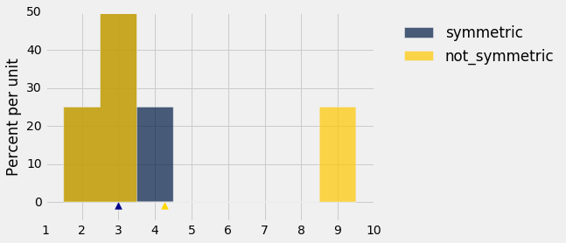### 示例

`sf2015`表包含 2015 年旧金山城市员工的薪水和福利数据。与以前一样，我们将我们的分析仅限于那些等价于至少就业半年的人。

``````sf2015 = Table.read_table('san_francisco_2015.csv').where('Salaries', are.above(10000))
``````

``````sf2015.select('Total Compensation').hist(bins = np.arange(10000, 700000, 25000))
``````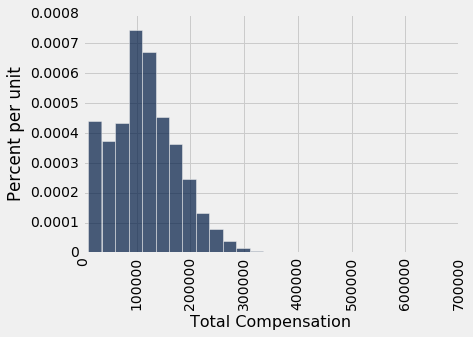``````compensation = sf2015.column('Total Compensation')
percentile(50, compensation)
110305.78999999999
np.mean(compensation)
114725.98411824222
``````

## 可变性

### 距离均值的偏差的大致大小

``````any_numbers = make_array(1, 2, 2, 10)
``````

``````# Step 1. The average.

mean = np.mean(any_numbers)
mean
3.75
``````

``````# Step 2. The deviations from average.

deviations = any_numbers - mean
calculation_steps = Table().with_columns(
'Value', any_numbers,
'Deviation from Average', deviations
)
calculation_steps
``````
Value Deviation from Average
1 -2.75
2 -1.75
2 -1.75
10 6.25

``````sum(deviations)
0.0
``````

``````np.mean(deviations)
0.0
``````

``````# Step 3. The squared deviations from average

squared_deviations = deviations ** 2
calculation_steps = calculation_steps.with_column(
'Squared Deviations from Average', squared_deviations
)
calculation_steps
``````
Value Deviation from Average Squared Deviations from Average
1 -2.75 7.5625
2 -1.75 3.0625
2 -1.75 3.0625
10 6.25 39.0625
``````# Step 4. Variance = the mean squared deviation from average

variance = np.mean(squared_deviations)
variance
13.1875
``````

``````# Step 5.
# Standard Deviation:    root mean squared deviation from average
# Steps of calculation:   5    4      3       2             1

sd = variance ** 0.5
sd
3.6314597615834874
``````

### 标准差

``````np.std(any_numbers)
3.6314597615834874
``````

### 使用 SD

``````nba13 = Table.read_table('nba2013.csv')
nba13
``````
Name Position Height Weight Age in 2013
DeQuan Jones Guard 80 221 23
Darius Miller Guard 80 235 23
Trevor Ariza Guard 80 210 28
James Jones Guard 80 215 32
Wesley Johnson Guard 79 215 26
Klay Thompson Guard 79 205 23
Thabo Sefolosha Guard 79 215 29
Chase Budinger Guard 79 218 25
Kevin Martin Guard 79 185 30
Evan Fournier Guard 79 206 20

（省略了 495 行）

``````nba13.select('Height').hist(bins=np.arange(68, 88, 1))
``````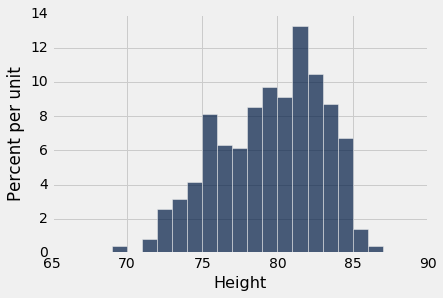NBA 球员身材高大并不奇怪！ 他们的平均身高只有 79 英寸（6'7"），比美国男子的平均身高高出 10 英寸。

``````mean_height = np.mean(nba13.column('Height'))
mean_height
79.065346534653472
``````

``````sd_height = np.std(nba13.column('Height'))
sd_height
3.4505971830275546
``````

``````nba13.sort('Height', descending=True).show(3)
``````
Name Position Height Weight Age in 2013
Hasheem Thabeet Center 87 263 26
Roy Hibbert Center 86 278 26
Tyson Chandler Center 85 235 30

（省略了 502 行）

Thabeet 比平均身高高了大约 8 英寸。

``````87 - mean_height
7.9346534653465284
``````

``````(87 - mean_height)/sd_height
2.2995015194397923
``````

``````nba13.sort('Height').show(3)
``````
Name Position Height Weight Age in 2013
Isaiah Thomas Guard 69 185 24
Nate Robinson Guard 69 180 29
John Lucas III Guard 71 157 30

（省略了 502 行）

``````(69 - mean_height)/sd_height
-2.9169868288775844
``````

### 使用 SD 度量延展度的最主要原因

``````nba13.select('Age in 2013').hist(bins=np.arange(15, 45, 1))
``````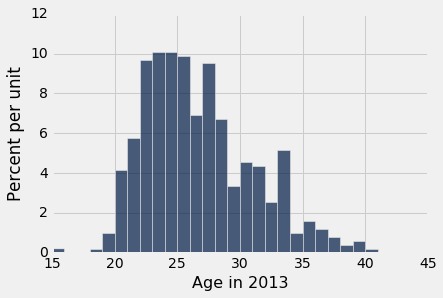``````ages = nba13.column('Age in 2013')
mean_age = np.mean(ages)
sd_age = np.std(ages)
mean_age, sd_age
(26.19009900990099, 4.3212004417203067)
``````

Juwan Howard 是年龄最大的球员 40 岁。

``````nba13.sort('Age in 2013', descending=True).show(3)
``````
Name Position Height Weight Age in 2013
Juwan Howard Forward 81 250 40
Marcus Camby Center 83 235 39
Derek Fisher Guard 73 210 39

（省略了 502 行）

Howard 的年龄比均值高了 3.2 个标准差。

``````(40 - mean_age)/sd_age
3.1958482778922357
``````

``````nba13.sort('Age in 2013').show(3)
``````
Name Position Height Weight Age in 2013
Jarvis Varnado Forward 81 230 15
Giannis Antetokounmpo Forward 81 205 18
Sergey Karasev Guard 79 197 19

（省略了 502 行）

``````(15 - mean_age)/sd_age
-2.5895811038670811
``````

### 标准单位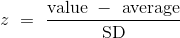``````def standard_units(numbers_array):
"Convert any array of numbers to standard units."
return (numbers_array - np.mean(numbers_array))/np.std(numbers_array)
``````

### 示例

``````united = Table.read_table('united_summer2015.csv')
united = united.with_column(
'Delay (Standard Units)', standard_units(united.column('Delay'))
)
united
``````
Date Flight Number Destination Delay Delay (Standard Units)
6/1/15 73 HNL 257 6.08766
6/1/15 217 EWR 28 0.287279
6/1/15 237 STL -3 -0.497924
6/1/15 250 SAN 0 -0.421937
6/1/15 267 PHL 64 1.19913
6/1/15 273 SEA -6 -0.573912
6/1/15 278 SEA -8 -0.62457
6/1/15 292 EWR 12 -0.117987
6/1/15 300 HNL 20 0.0846461
6/1/15 317 IND -10 -0.675228

（省略了 13815 行）

``````united.sort('Delay', descending=True)
``````
Date Flight Number Destination Delay Delay (Standard Units)
6/21/15 1964 SEA 580 14.269
6/22/15 300 HNL 537 13.1798
6/20/15 353 ORD 505 12.3693
8/23/15 1589 ORD 458 11.1788
7/23/15 1960 LAX 438 10.6722
6/23/15 1606 ORD 430 10.4696
6/4/15 1743 LAX 408 9.91236
6/17/15 1122 HNL 405 9.83637
7/27/15 572 ORD 385 9.32979

（省略了 13815 行）

``````within_3_sd = united.where('Delay (Standard Units)', are.between(-3, 3))
within_3_sd.num_rows/united.num_rows
0.9790235081374322
``````

``````united.hist('Delay (Standard Units)', bins=np.arange(-5, 15.5, 0.5))
plots.xticks(np.arange(-6, 17, 3));
``````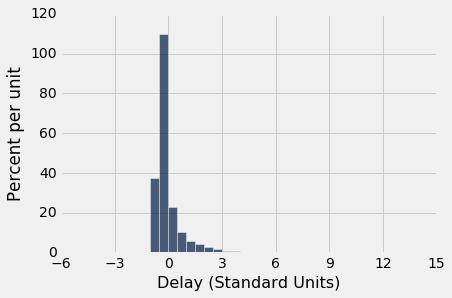## 标准差和正态曲线

### 数据的大致钟形的直方图

``````baby = Table.read_table('baby.csv')
heights = baby.column('Maternal Height')
mean_height = np.round(np.mean(heights), 1)
mean_height
64.0
sd_height = np.round(np.std(heights), 1)
sd_height
2.5
baby.hist('Maternal Height', bins=np.arange(55.5, 72.5, 1), unit='inch')
positions = np.arange(-3, 3.1, 1)*sd_height + mean_height
plots.xticks(positions);
``````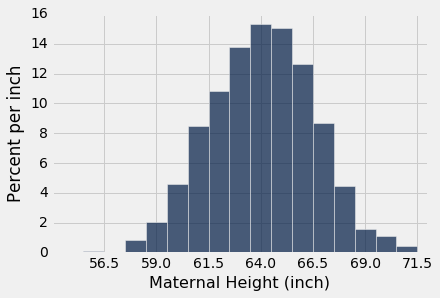### 标准正态曲线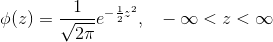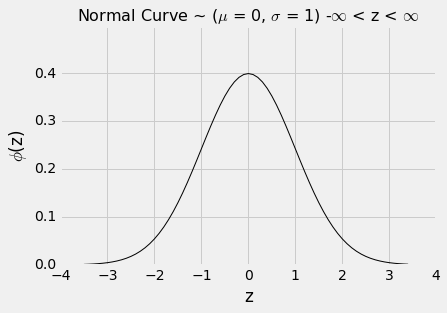``````from scipy import stats
``````

### 标准正态的累积分布函数（CDF）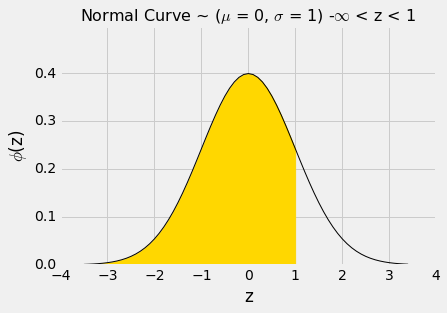``````stats.norm.cdf(1)
0.84134474606854293
``````

`z = 1`右侧的面积大概是`100% - 84% = 16%`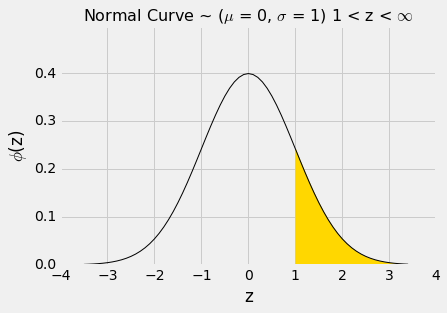``````1 - stats.norm.cdf(1)
0.15865525393145707
``````

`z = -1``z = 1`之间的面积可以用几种不同的方式来计算。 它是下面的曲线下方的金色区域。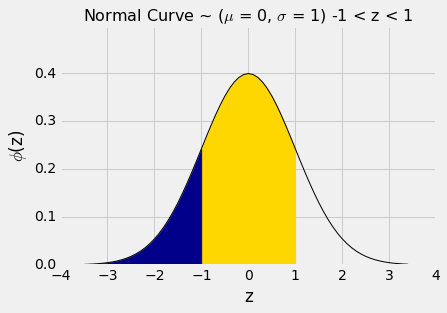``````stats.norm.cdf(1) - stats.norm.cdf(-1)
0.68268949213708585
````````````stats.norm.cdf(2) - stats.norm.cdf(-2)
0.95449973610364158
``````

Percent in Range All Distributions: Bound Normal Distribution: Approximation

## 中心极限定律

### 轮盘赌的净收益

``````wheel
``````
Pocket Color
0 green
00 green
1 red
2 black
3 red
4 black
5 red
6 black
7 red
8 black

（省略了 28 行）

``````def red_winnings(color):
if color == 'red':
return 1
else:
return -1
``````

`red`表展示了红色情况下，每个口袋的奖金。

``````red = wheel.with_column(
'Winnings: Red', wheel.apply(red_winnings, 'Color')
)
red
``````
Pocket Color Winnings: Red
0 green -1
00 green -1
1 red 1
2 black -1
3 red 1
4 black -1
5 red 1
6 black -1
7 red 1
8 black -1

（省略了 28 行）

``````red.select('Winnings: Red').hist(bins=np.arange(-1.5, 1.6, 1))
``````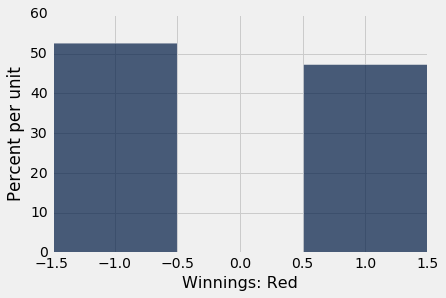``````num_bets = 400
repetitions = 10000

net_gain_red = make_array()

for i in np.arange(repetitions):
spins = red.sample(num_bets)
new_net_gain_red = spins.column('Winnings: Red').sum()
net_gain_red = np.append(net_gain_red, new_net_gain_red)

results = Table().with_column(
'Net Gain on Red', net_gain_red
)
results.hist(bins=np.arange(-80, 50, 6))
``````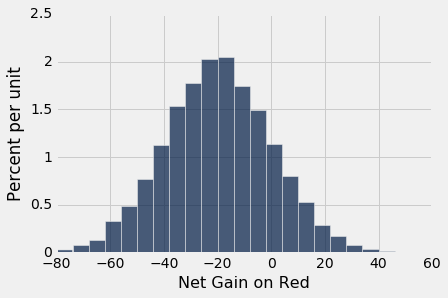``````average_per_bet = 1*(18/38) + (-1)*(20/38)
average_per_bet
-0.05263157894736842
``````

``````400 * average_per_bet
-21.052631578947366
``````

``````np.mean(results.column(0))
-20.8992
``````

``````np.std(results.column(0))
20.043159415621083
``````

### 平均航班延误

`united`表包含 2015 年夏季旧金山机场出发的 13,825 个联合航空国内航班的出发延误数据。正如我们以前所见，延误的分布的右侧有着很长的尾巴。

``````united = Table.read_table('united_summer2015.csv')
united.select('Delay').hist(bins=np.arange(-20, 300, 10))
``````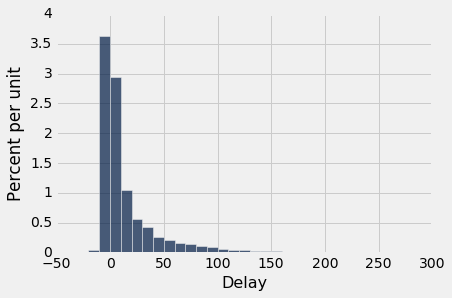``````mean_delay = np.mean(united.column('Delay'))
sd_delay = np.std(united.column('Delay'))

mean_delay, sd_delay
(16.658155515370705, 39.480199851609314)
``````

``````delay = united.select('Delay')
np.mean(delay.sample(400).column('Delay'))
16.68
``````

``````sample_size = 400
repetitions = 10000

means = make_array()

for i in np.arange(repetitions):
sample = delay.sample(sample_size)
new_mean = np.mean(sample.column('Delay'))
means = np.append(means, new_mean)

results = Table().with_column(
'Sample Mean', means
)
results.hist(bins=np.arange(10, 25, 0.5))
``````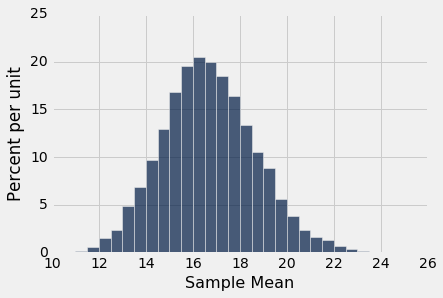### 紫色的花的分布

``````colors = make_array('Purple', 'Purple', 'Purple', 'White')

model = Table().with_column('Color', colors)

model
``````
Color
Purple
Purple
Purple
White
``````props = make_array()

num_plants = 200
repetitions = 10000

for i in np.arange(repetitions):
sample = model.sample(num_plants)
new_prop = np.count_nonzero(sample.column('Color') == 'Purple')/num_plants
props = np.append(props, new_prop)

results = Table().with_column('Sample Proportion: 200', props)
results.hist(bins=np.arange(0.65, 0.85, 0.01))
````````````props2 = make_array()

num_plants = 800

for i in np.arange(repetitions):
sample = model.sample(num_plants)
new_prop = np.count_nonzero(sample.column('Color') == 'Purple')/num_plants
props2 = np.append(props2, new_prop)

results = results.with_column('Sample Proportion: 800', props2)
results.hist(bins=np.arange(0.65, 0.85, 0.01))
``````## 样本均值的可变性

``````united = Table.read_table('united_summer2015.csv')
delay = united.select('Delay')
pop_mean = np.mean(delay.column('Delay'))
pop_mean
16.658155515370705
``````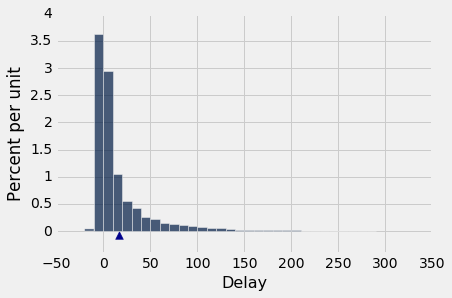``````"""Empirical distribution of random sample means"""

def simulate_sample_mean(table, label, sample_size, repetitions):

means = make_array()

for i in range(repetitions):
new_sample = table.sample(sample_size)
new_sample_mean = np.mean(new_sample.column(label))
means = np.append(means, new_sample_mean)

sample_means = Table().with_column('Sample Means', means)

# Display empirical histogram and print all relevant quantities
sample_means.hist(bins=20)
plots.xlabel('Sample Means')
plots.title('Sample Size ' + str(sample_size))
print("Sample size: ", sample_size)
print("Population mean:", np.mean(table.column(label)))
print("Average of sample means: ", np.mean(means))
print("Population SD:", np.std(table.column(label)))
print("SD of sample means:", np.std(means))
``````

``````simulate_sample_mean(delay, 'Delay', 100, 10000)
plots.xlim(5, 35)
plots.ylim(0, 0.25);
Sample size:  100
Population mean: 16.6581555154
Average of sample means:  16.662059
Population SD: 39.4801998516
SD of sample means: 3.90507237968
``````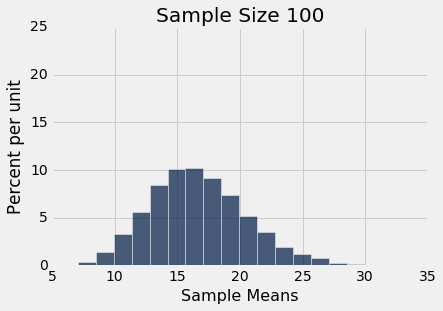``````simulate_sample_mean(delay, 'Delay', 400, 10000)
plots.xlim(5, 35)
plots.ylim(0, 0.25);
Sample size:  400
Population mean: 16.6581555154
Average of sample means:  16.67117625
Population SD: 39.4801998516
SD of sample means: 1.98326299651
``````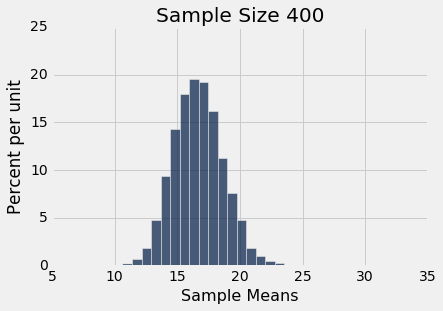``````simulate_sample_mean(delay, 'Delay', 625, 10000)
plots.xlim(5, 35)
plots.ylim(0, 0.25);
Sample size:  625
Population mean: 16.6581555154
Average of sample means:  16.68523712
Population SD: 39.4801998516
SD of sample means: 1.60089096006
``````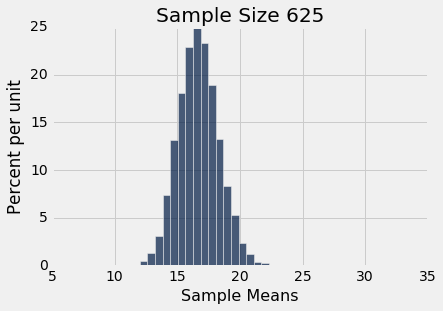### 所有样本均值的 SD

``````pop_sd = np.std(delay.column('Delay'))
pop_sd
39.480199851609314
``````

``````repetitions = 10000
sample_sizes = np.arange(25, 626, 25)

sd_means = make_array()

for n in sample_sizes:
means = make_array()
for i in np.arange(repetitions):
means = np.append(means, np.mean(delay.sample(n).column('Delay')))
sd_means = np.append(sd_means, np.std(means))

sd_comparison = Table().with_columns(
'Sample Size n', sample_sizes,
'SD of 10,000 Sample Means', sd_means,
'pop_sd/sqrt(n)', pop_sd/np.sqrt(sample_sizes)
)
sd_comparison
``````
Sample Size n SD of 10,000 Sample Means pop_sd/sqrt(n)
25 7.95017 7.89604
50 5.53425 5.58334
75 4.54429 4.55878
100 3.96157 3.94802
125 3.51095 3.53122
150 3.23949 3.22354
175 3.00694 2.98442
200 2.74606 2.79167
225 2.63865 2.63201
250 2.51853 2.49695

（省略了 15 行）

``````sd_comparison.plot('Sample Size n')
``````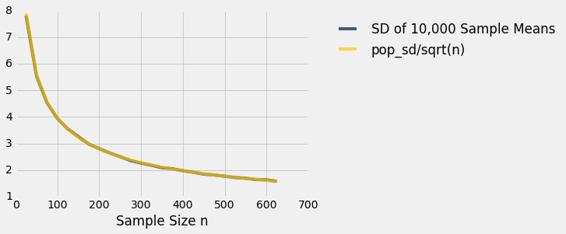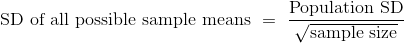### 样本均值的准确性

• 总体大小不影响样本均值的准确性。公式中的任何地方都没有出现总体大小。
• 总体标准差是一个常数；从总体中抽取的每个样本都是一样的。样本量可以变化。由于样本量出现在分母中，样本均值的可变性随着样本量的增加而降低，因此准确度增加。

## 选取样本量

• 选民人数非常多，所以我们可以假定随机样本带放回地抽取。
• 投票机构将通过为候选人 A 的选民百分比，构建一个约 95% 置信区间来做出估计。
• 准确度的理想水平是间隔宽度不应超过 1%。这非常准确！例如，置信区间`(33.2%, 34%)`可以，但`(33.2％, 35％)`不行。
• 我们将以候选人 A 的选民比例为例。回想一下，比例是一个平均值，其中总体中的值只有 0（你不计算的个体类型）或 1（你计算的个体类型）。

### 置信区间的宽度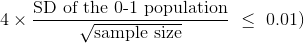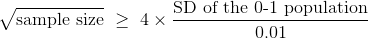### 01 集合的标准差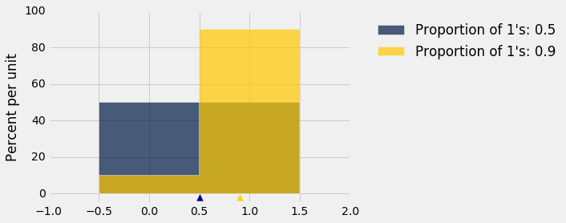``````sd = make_array()
for i in np.arange(1, 10, 1):
# Create an array of i 1's and (10-i) 0's
population = np.append(np.ones(i), 1-np.ones(10-i))
sd = np.append(sd, np.std(population))

zero_one_sds = Table().with_columns(
"Population Proportion of 1's", np.arange(0.1, 1, 0.1),
"Population SD", sd
)

zero_one_sds
``````
Population Proportion of 1's Population SD
0.1 0.3
0.2 0.4
0.3 0.458258
0.4 0.489898
0.5 0.5
0.6 0.489898
0.7 0.458258
0.8 0.4
0.9 0.3

``````zero_one_sds.scatter("Population Proportion of 1's")
``````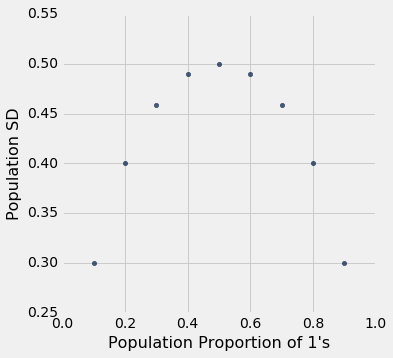### 样本量